GeeksforGeeks App
Open AppBrowser
Continue

# Perimeter Formulas for Geometric Shapes

In mathematics or in daily lives geometry and geometrical shapes always play an essential role. Starting from a simple tray to a big building, we are always surrounded by geometry in different shapes and sizes. The shape and surface to be taken up by such shapes need to be calculated so as to store them without any difficulty, or to meet the needs of the people using them. Such study or computation is given a special name in the field of mathematics.

Whenever the dimensions of various geometrical shapes are computed and their area or capacity is measured in mathematics, it is termed mensuration. Mensuration also helps to calculate the dimensions of other shapes from their surface area and capacity.

Perimeter can be defined as the boundary of any closed figure. Perimeter is the measure of all sides of any closed figure say a triangle, rectangle, square, circle etc.

General formula for perimeter of any closed figure = Sum of all sides

Now lets find the perimeter formula for various shapes.

### Perimeter of a Triangle

Triangle is a closed figure which is formed by three straight lines. These lines forms sides of a triangle so it has three sides say A, B, C. These three sides can be same or different depending on the type of triangle.

Types of triangles

There are three types of triangles: Scalene, Isosceles, Equilateral.

Scalene Triangle: The triangle in which all three sides are different is called the scalene triangle.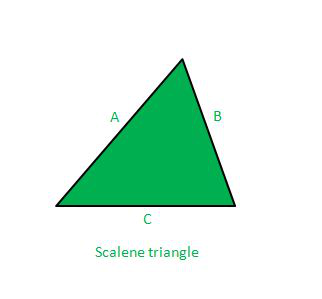Perimeter of Scalene Triangle = A + B + C

Isosceles Triangle: The triangle in which two sides are equal is called the Isosceles triangle.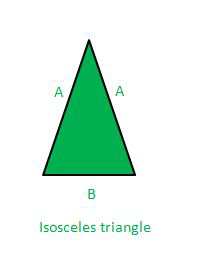Here two sides are equal.

Perimeter of Isosceles Triangle = A + A + B  = 2 × A + B

Equilateral Triangle: The triangle in which all three sides are equal is called an Equilateral triangle.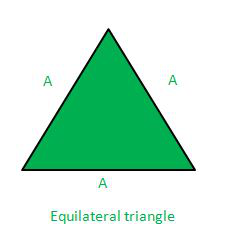Here all three sides are equal.

Perimeter of Equilateral Triangle = A + A + A = 3×A

### Square

Square is a closed figure which is made by four straight lines. All four lines are equal and all four angles of a square are of 90 degrees.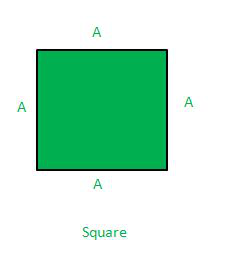Perimeter of Square = Sum of all 4 sides =  4×A

### Perimeter of Rectangle

A rectangle is a closed figure which is made by four straight lines. Both pairs of opposite lines are equal and all four angles of a rectangle are of 90 degrees.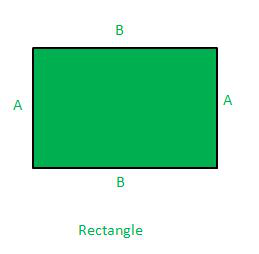Perimeter of Rectangle = Sum of all sides = 2×A + 2×B = 2(A + B)

### Perimeter of Circle

Circle is a closed figure whose boundary is at a constant distance from the centre of the circle. This constant distance from centre of circle to boundary is called as radius of circle.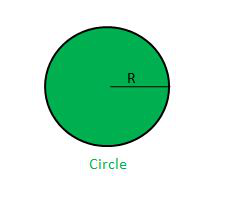Perimeter of circle also known as Circumference of circle = 2×π×R

### Sample Questions

Question 1: What is the perimeter of triangle which has sides a = 5 cm, b = 8 cm, c = 10 cm?

As all sides of the given triangle are different so it is a scalene triangle, hence perimeter of scalene triangle is sum of all sides.

Perimeter = a + b + c = 5 + 8 + 10

Perimeter = 23 cm

Question 2: What is the perimeter of a circle whose radius is 7 cm?

Perimeter of a circle is given by formula 2×π×R

So perimeter = 2 × (22/7) × 7                  value of π is 22/7

= 44 cm

Question 3: What is the perimeter of square which has each side equals to 10 cm?

Perimeter of a square is given by the sum of all sides.

Perimeter = 4 × Side

= 4 × 10

= 40 cm

Question 4: What is the perimeter of a rectangle whose length = 5 cm and breadth = 8 cm?

Perimeter of rectangle is given by formula = 2×(A + B) where A is length and B is breadth.

Perimeter = 2 × (A + B)

= 2 × (5 + 8)

= 2 × (13)

= 26 cm

Question 5: What is the perimeter of an equilateral triangle whose side is 8 cm?